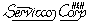Sampling Plans Site Map
 Main Areas:Software Area: [ Acceptance sampling software ] [ Audit sampling software ] [ How to Buy Software ] [ Specifications & Support ] Acceptance Sampling:  [ TPZero - Tool for Ac=0 Plans ] [ TPZero - Screen Shots ] [ TP105 - Software for Attributes ] [ Binomial Examples ] [ Poisson Examples ] [ TP414 - Software for Variables ] [ ISL Examples ] [ Lot Mean Examples ] [ TP781 - Software for Reliability ]Binomial Examples of TP105 Sampling Plans for Attribute Data visitors=Decision Rules
Fixed-n decision rule
Sequential decision rule: n-table
Sequential decision rule: x-table
Sequential decision rule diagram (text)
Sequential decision rule exported & displayed as an MS Excel chart
Sequential slope and intercept
Performance Curves (for matched fixed-n & sequential plans)
Performance curve tables by Pa
Performance curve tables by p'
OC-Curve graph (text)
OC-Curve graph exported & displayed as an MS Excel chart
AOQ-Curve graph exported & displayed as an MS Excel chart
ASN-Curve graph exported & displayed as an MS Excel chart
ARL-Curve graph exported & displayed as an MS Excel chartFixed-n Decision Rule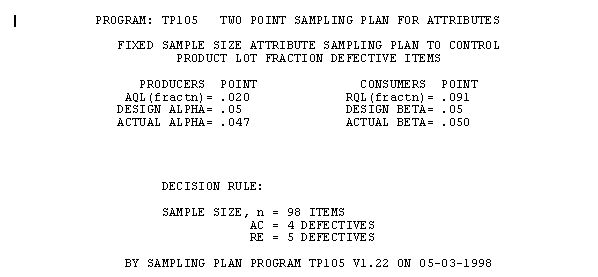The sampling plan documents the type of plan, the two points on the oc-curve that specified the plan, the decision rule, and the date and version of the software.Sequential Decision Rule:  n-TableThe n-table refers that you enter the table with the left two sample size (n) columns.Sequential Decision Rule: X-Table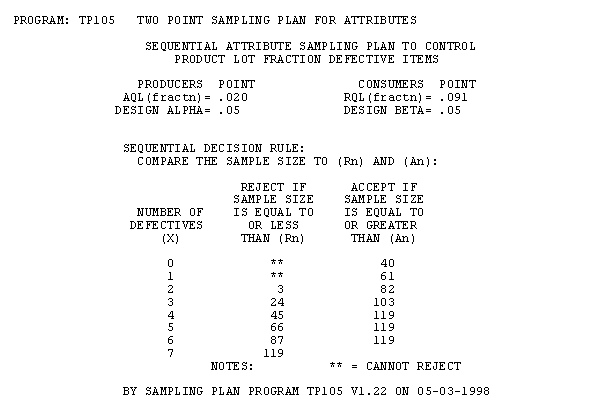The x-table refers that you enter the table with the left defectives (x) column.Sequential Decision Diagram (text)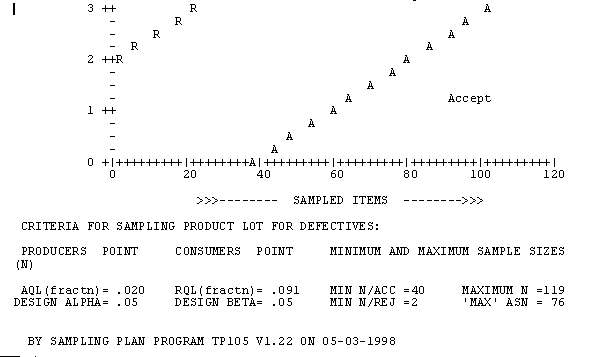The minimum and maximum sample sizes shown in the reports footer above can be verified by the diagram. The minimum n to accept = 40 occurs when the first 40 sampled items are non-defective -- where the acceptance line intersects the x-axis. The minimum n to reject =2 occurs when the first two sampled items are defective -- where the rejection line intersects the n=1. The maximum n = 119 is the right-hand truncation of the continue zone. The truncation is user-controlled but otherwise defaults to a good-practice value. The 'maximum' ASN =76 is the maximum of the ASN curve.Sequential Sampling Plan from TP105 Displayed as an MS Excel Chart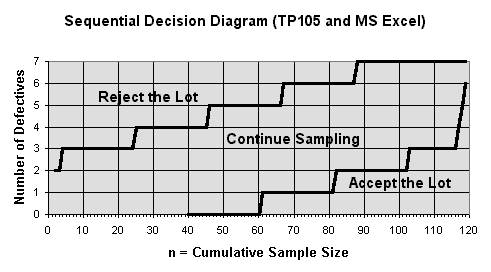Plot the data on the diagram sequentially as it is collected. The accept or reject decision is made when sample data plots on or over an acceptance line or rejection line.The stair-step pattern is caused by the grouping that occurs in the sequential decision n-table upon which this diagram is based. (previous-above). This is natural with attribute data because the y-axis, number of defectives, is always a whole number.Slope and Intercept of Sequential Lines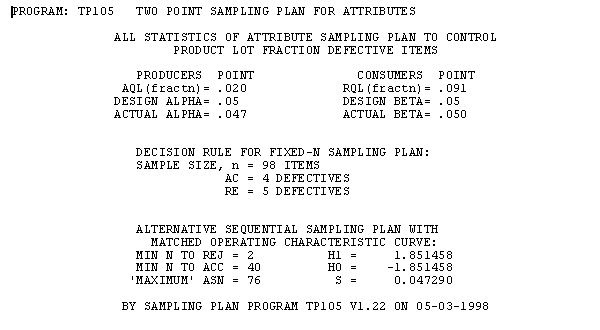This report provides the slope and intercepts of the parallel acceptance and rejection lines of the sequential sampling plan who's oc-curve matches that of the fixed-n curve shown.Performance Curve Tables by Pa -- Matched Fixed-n & Sequential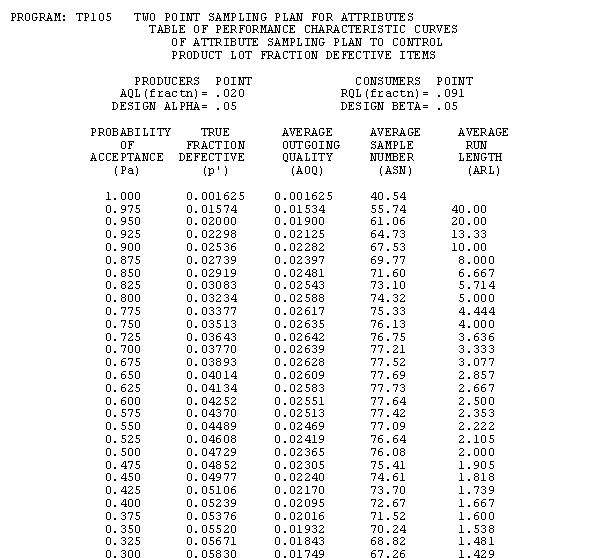The left column (Pa) has constant step-size.Performance Curve Tables by p' -- Matched Fixed-n & Sequential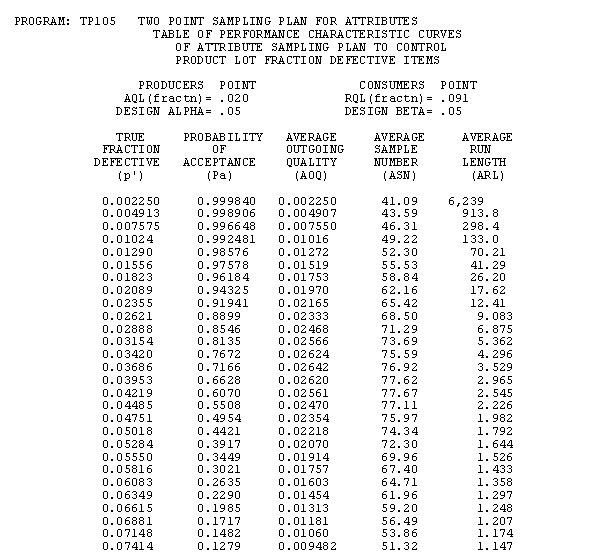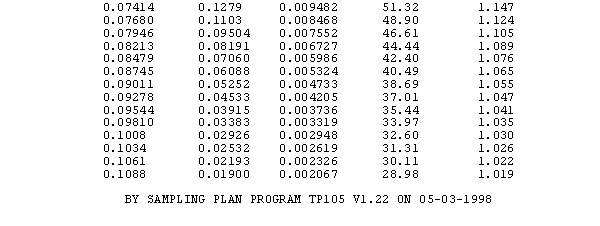The left column (p') has constant step-size. This table was used to build the four MS Excel charts below (OC, AOQ, ASN, and ARL)Operating Characteristic Curve Graph (text)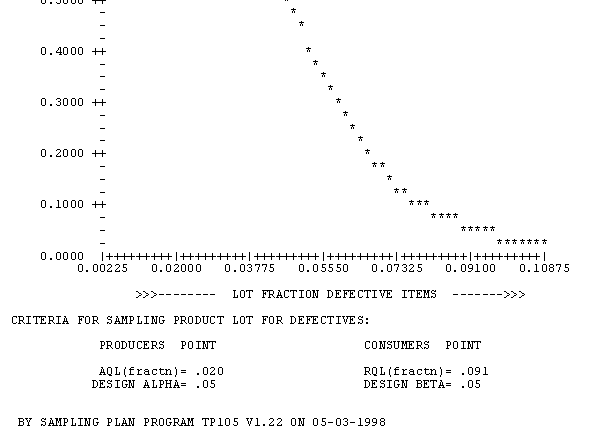In most cases, this text graph is sufficient to evaluate and/or compare sampling plans.OC-Curve from TP105 Displayed as MS Excel Chart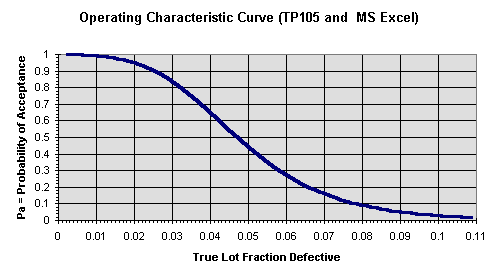This chart was made with the MS Excel chart wizard after writing the TP105 performance table to a file, importing it into Excel, and using [data][text to columns] in Excel to parse the tabular data into columns. This procedure has been tested with Excel 5.0 and 7.0(7.0 is office 95)AOQ-Curve from TP105 Displayed as MS Excel Chart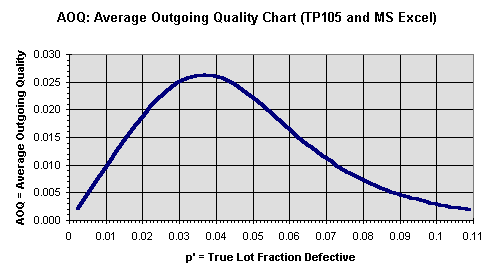This AOQ curve is based on AOQ = Pa * p'. It is not adjusted for lot size.ASN-Curve from TP105 Displayed as MS Excel Chart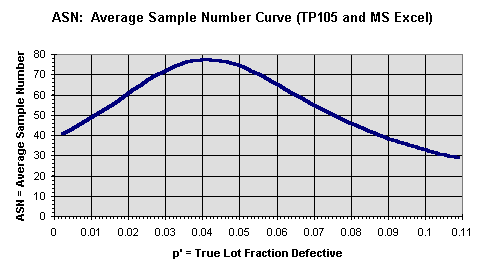The ASN-Curve is useful to estimate the sample size that will be required by a sequential sampling plan for various lot fractions defective.ARL-Curve from TP105 Displayed as MS Excel Chart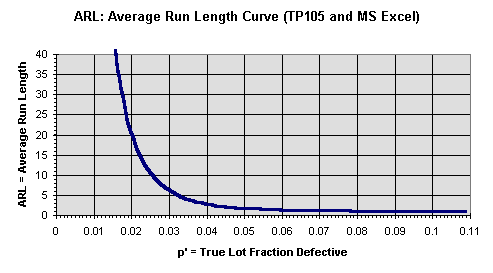The ARL is the average number of lot acceptances (a run) before a lot is rejected. You want a high ARL at the producer's point and a low ARL at the consumer's point.